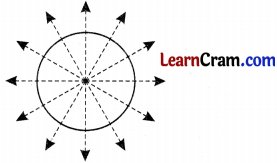# DAV Class 7 Maths Chapter 13 Worksheet 1 Solutions

The DAV Class 7 Maths Book Solutions and DAV Class 7 Maths Chapter 13 Worksheet 1 Solutions of Symmetry offer comprehensive answers to textbook questions.

## DAV Class 7 Maths Ch 13 WS 1 Solutions

Question 1.
Make any two ink-dot designs.
Take a piece of paper and put some ink drops on it. Now, fold the paper and press two parts against each other. You will get ink-drt design.Question 2.
Make any two symmetrical paper cut designs.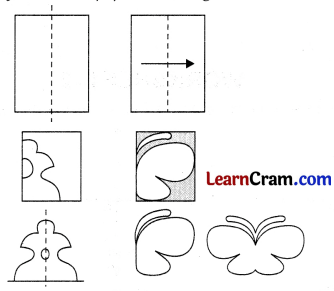Question 3.
Mark the corresponding holes for the given line of symmetry in the following figures.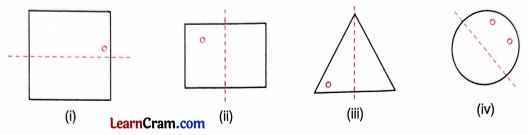Question 4.
Write three letters of English alphabet each having the following number of lines of symmetry,
(i) one line of symmetry.(ii) two lines of symmetry.Question 5.
Write five letters of English alphabet having no line of symmetry.
F, G, N, P, Q, J, K, S, Z

Question 6.
Draw the line of symmetry for the following figures.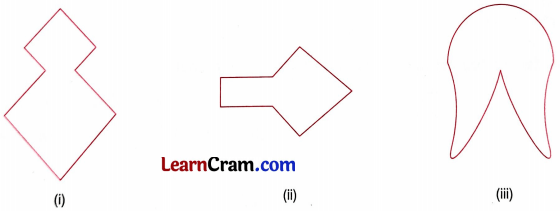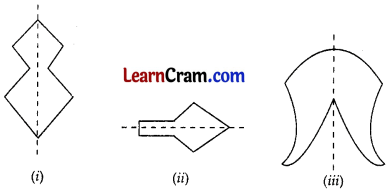### DAV Class 8 Maths Chapter 13 Worksheet 1 Notes

A figure is said to be symmetrical about a line if it is folded about that line, the two part cover completely each other.

Example: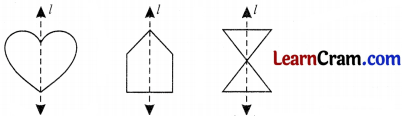The above figures are symmetrical about the line l.

The line of symmetry of a line segment is perpendicular bisector of the line.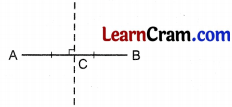A line has infinitely many lines of symmetry.

The line of symmetry of an angle is the bisector of the angle itself.A regular polygon of n sides has i lines of symmetry.
There are infinite number of lines of symmetry of a circle. Infact each diameter of a circle its line of symmetry.